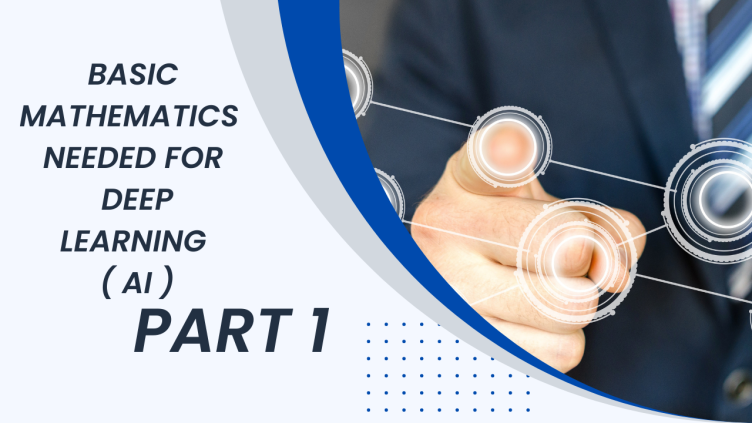# BASIC MATHEMATICS FOR DEEP LEARNING (AI) PART 2 - FUNCTION, GRAPHS, LINEAR EQUATIONS

Deep learning is numerical computational programming some of them also called it a differential programming. In this type of programming we don't write lengthy codes instead we provide basic mathematical formula which do that lengthy codes job. So for learning deep learning we need to first learn basic mathematics . In my previous post we have explore vectors, Means, Matrices and standard deviation. In this post we will explore Functions,Graph, Linear Equation and one theorem which is called universal approximation theorem and will provide python code which you can run and feel of working those mathematical formula.

## FUNCTION

Technically speaking a function is a relation between input and output. It is like a machine which converts your input to some other output and that output is related somehow to that input. Function is written as (y=f(x)) in mathematics, where F represents that relationships and x represent input and y represent output.

We have only three things in function one is input, output and relationship (y=f(x))

It is also represent as below

All possible input are called domain and all possible output after passing through that relation is called range.

A function can also be thought as collection of some input is some fashion which give's you some output. Let's take on example Jon father is 25 times older than Jon. Then if Jon's age 15 what is his fathers age. This can be represented as a function.

FATHER'S AGE = 25 + JON AGE

JONS AGE = 15

FATHERS AGE = 25+15=40

In the above example we can consider like this that Jons age is a independent variable and Jons father age is dependent variable which is dependent on Jons age, so here input is Jon's age and output is his father's age and relationship function between them is 25.

Like wise a function is a relationship between two variables.

Function in mathematics is a way of representing idea of relationships between two things in this world. If one thing is dependent on other then that thing can be represented by function. Let say in deep learning dogs picture is there which related to dog text. so dog text is related to dog image can be expressed in the function. Finding that function is a job of deep learning.

PYTHON CODE for Jons example

JON = 15

FATHER = 25+JON

PRINT(FATHER)

## GRAPH

Graph is a visual representation of function on paper where y coordinates, represent dependent variable and x coordinates represent independent variable. For our Jons example graph will be represented as

As Jon's grew older jons father grew 25 years ahead.

Like wise we have many redefined function which has its graph representation. This function have very much importance in physical science's, such function are sine, cosine, tanh, quandratic function, polynomial function and many more. You can find more details about it only in deep learning. We mainly use linear function and its derivatives for learning purpose. In optimizer and loss functions we use many other functions

Graph drawing in python using MATHPLOTLIB for Jon's sage example is given below import mathplotlib.

## LINEAR EQUATION

Deep learning highly uses linear equation in learning representation of input and provides it output. Linear equation is basically a function which relates input to output linearly with some intercepts. If some input is directly related to its output , Jon's father age is clear example of linear equation.

FATHER'S = JON'S+25

Here above father is linear associated with jon's age plus 25, here 25 is intercept. Any equation of this type

y=ax+b

is example of linear equation, where a is called slope and b is called intercept, here in above Jon's example 1 is slope and 25 is intercept. In linear equation if one input changes than output variable will also be changed. Its graph represent will be like

We uses linear equation in deep learning in learning weights. In deep learning we consider slope as weights and intercept as bias.

## UNIVERSAL APPROXIMATION THEOREM

UNIVERSAL APPROXIMATION THEOREM state that anything in this world can be defined and expressed by function. You can approximate anything in this world through function. This theorem is stated long back ago but none of the technology at that times supports it's full strength. But after invention of deep learning by Geoffrey Hinton and his team. This theorem is totally satisfied. Deep learning performs this theorem. It is stated that when linear equation is passed through some activation than take its derivative will provide you such weight which can act as a function. The input representation means a function can represent anything in this world. Lets say you have dog image and dog as a label in text. here above dog image is a input and label is a output to that image we pass this image in linear function than pass its through activation and its derivation. After taking derivatives and adding those gradient to original function will give you function which correlate that input image of dog to output text dog. Its little bit confusing but you will get it as you go.

## CONCLUSION

We have discussed different mathematical concept which are required to learn deep learning (AI). We have discussed Function, Graph, Linear Equation and highly important universal approximation theorem. In next blog we will discuss more about derivatives and how much framework provide as library to form derivatives.